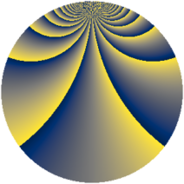# Properties

 Label 3549.2.cuLevel $3549$ Weight $2$ Character orbit 3549.cu Rep. character $\chi_{3549}(34,\cdot)$ Character field $\Q(\zeta_{52})$ Dimension $5856$ Sturm bound $970$

# Related objects

## Defining parameters

 Level: $$N$$ $$=$$ $$3549 = 3 \cdot 7 \cdot 13^{2}$$ Weight: $$k$$ $$=$$ $$2$$ Character orbit: $$[\chi]$$ $$=$$ 3549.cu (of order $$52$$ and degree $$24$$) Character conductor: $$\operatorname{cond}(\chi)$$ $$=$$ $$1183$$ Character field: $$\Q(\zeta_{52})$$ Sturm bound: $$970$$

## Dimensions

The following table gives the dimensions of various subspaces of $$M_{2}(3549, [\chi])$$.

Total New Old
Modular forms 11712 5856 5856
Cusp forms 11520 5856 5664
Eisenstein series 192 0 192

## Trace form

 $$5856q - 4q^{7} + 488q^{9} + O(q^{10})$$ $$5856q - 4q^{7} + 488q^{9} - 16q^{11} + 32q^{14} + 512q^{16} - 12q^{21} - 4q^{28} + 480q^{32} + 16q^{35} + 24q^{39} + 24q^{42} - 16q^{44} + 8q^{46} - 40q^{50} - 40q^{57} - 48q^{58} + 16q^{60} + 4q^{63} - 16q^{65} - 360q^{67} - 192q^{70} - 24q^{71} - 384q^{74} - 32q^{78} - 488q^{81} - 36q^{84} + 456q^{85} + 576q^{86} - 36q^{91} - 144q^{92} - 40q^{93} - 120q^{98} + 16q^{99} + O(q^{100})$$

## Decomposition of $$S_{2}^{\mathrm{new}}(3549, [\chi])$$ into newform subspaces

The newforms in this space have not yet been added to the LMFDB.

## Decomposition of $$S_{2}^{\mathrm{old}}(3549, [\chi])$$ into lower level spaces

$$S_{2}^{\mathrm{old}}(3549, [\chi]) \cong$$ $$S_{2}^{\mathrm{new}}(1183, [\chi])$$$$^{\oplus 2}$$